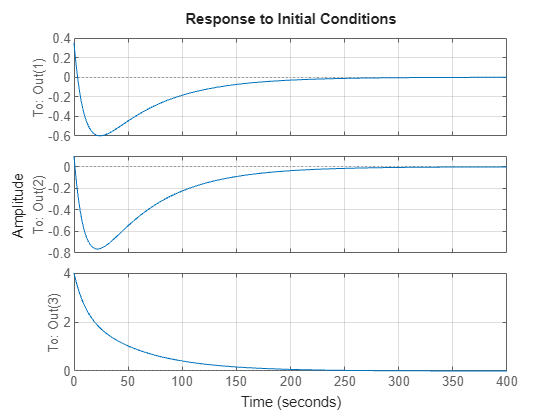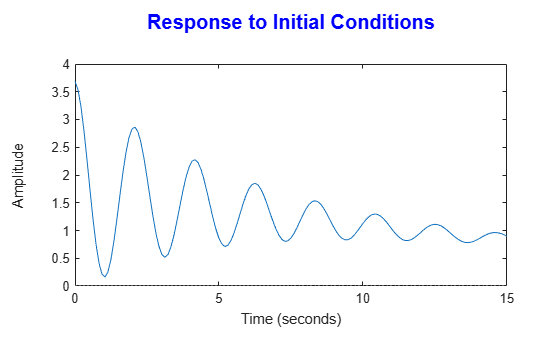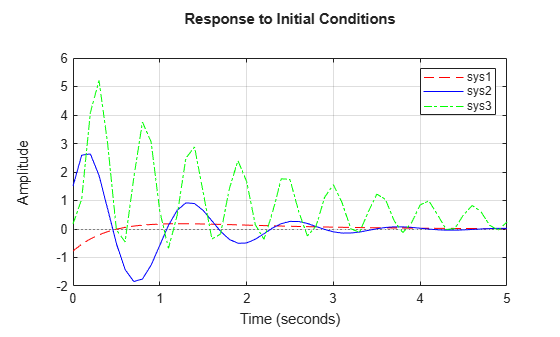# initialplot

Plot initial condition response with additional plot customization options

## Syntax

``h = initialplot(sys,x0)``
``h = initialplot(sys1,sys2,...,sysN,x0)``
``h = initialplot(sys1,LineSpec1,...,sysN,LineSpecN,x0)``
``h = initialplot(___,tFinal)``
``h = initialplot(___,t)``
``h = initialplot(AX,___)``
``h = initialplot(___,plotoptions)``

## Description

`initialplot` lets you plot initial condition responses with a broader range of plot customization options than `initial`. You can use `initialplot` to obtain the plot handle and use it to customize the plot, such as modify the axes labels, limits and units. You can also use `initialplot` to plot initial condition response on an existing set of axes represented by an axes handle. To customize an existing plot using the plot handle:

1. Obtain the plot handle

2. Use `getoptions` to obtain the option set

3. Update the plot using `setoptions` to modify the required options

For more information, see Customizing Response Plots from the Command Line. To create initial condition response plots with default options or to extract initial condition response data, use `initial`.

example

````h = initialplot(sys,x0)` plots the initial condition response of states of the dynamic system model `sys` and returns the plot handle `h` to the plot. You can use this handle `h` to customize the plot with the `getoptions` and `setoptions` commands.```

example

````h = initialplot(sys1,sys2,...,sysN,x0)` plots the initial condition response of multiple dynamic systems `sys1,sys2,…,sysN` on the same plot. All systems must have the same number of inputs and outputs to use this syntax.```

example

````h = initialplot(sys1,LineSpec1,...,sysN,LineSpecN,x0)` sets the line style, marker type, and color for the initial condition response of each system. All systems must have the same number of inputs and outputs to use this syntax.```

example

````h = initialplot(___,tFinal)` simulates the response of initial conditions from `t = 0` to the final time `t = tFinal`. Specify `tFinal` in the system time units, specified in the `TimeUnit` property of `sys`. For discrete-time systems with unspecified sample time `(Ts = -1)`, `initialplot` interprets `tFinal` as the number of sampling intervals to simulate.```

example

````h = initialplot(___,t)` simulates the response of initial conditions using the time vector `t`. Specify `t` in the system time units, specified in the `TimeUnit` property of `sys`.```
````h = initialplot(AX,___)` plots the initial condition response of the states on the `Axes` object in the current figure with the handle `AX`.```

example

````h = initialplot(___,plotoptions)` plots the response of the initial conditions with the options set specified in `plotoptions`. You can use these options to customize the plot appearance using the command line. Settings you specify in `plotoptions` overrides the preference settings in the MATLAB® session in which you run `initialplot`. Therefore, this syntax is useful when you want to write a script to generate multiple plots that look the same regardless of the local preferences.```

## Examples

collapse all

For this example, use the plot handle to change the time units to minutes and turn on the grid.

Generate a random state-space model with 5 states and create the initial conditon response plot with plot handle `h`.

```rng("default") sys = rss(5); x0 = [1,2,3,4,5]; h = initialplot(sys,x0);```Change the time units to minutes and turn on the grid. To do so, edit properties of the plot handle, `h` using `setoptions`.

`setoptions(h,'TimeUnits','minutes','Grid','on');`The plot automatically updates when you call `setoptions`.

Alternatively, you can also use the `timeoptions` command to specify the required plot options. First, create an options set based on the toolbox preferences.

`plotoptions = timeoptions('cstprefs');`

Change properties of the options set by setting the time units to minutes and enabling the grid.

```plotoptions.TimeUnits = 'minutes'; plotoptions.Grid = 'on'; h = initialplot(sys,x0,plotoptions);```You can use the same option set to create multiple initial condition plots with the same customization. Depending on your own toolbox preferences, the plot you obtain might look different from this plot. Only the properties that you set explicitly, in this example `TimeUnits` and `Grid`, override the toolbox preferences.

Consider the following two-input, two-output dynamic system.

`$sys\left(s\right)=\left[\begin{array}{cc}0& \frac{3s}{{s}^{2}+s+10}\\ \frac{s+1}{s+5}& \frac{2}{s+6}\end{array}\right].$`

Convert the `sys` to state-space form since initial condition plots are supported only for state-space models.

```sys = ss([0, tf([3 0],[1 1 10]) ; tf([1 1],[1 5]), tf(2,[1 6])]); size(sys)```
```State-space model with 2 outputs, 2 inputs, and 4 states. ```

The resultant state-space model has four states. Hence, provide an initial condition vector with four elements.

`x0 = [0.3,0.25,1,4];`

Use `timeoptions` to create a plot options set and turn the grid on. Provide an appropriate title before creating the initial conditions plot.

```plotoptions = timeoptions; plotoptions.Grid = 'on'; plotoptions.Title.String = 'Initial Condition Plot of MIMO System sys(s)'; h = initialplot(sys,x0,plotoptions);```For this example, consider a MIMO state-space model with 3 inputs, 3 outputs and 3 states. Create an initial condition plot with red colored grid lines.

Create the MIMO state-space model `sys_mimo`.

```J = [8 -3 -3; -3 8 -3; -3 -3 8]; F = 0.2*eye(3); A = -J\F; B = inv(J); C = eye(3); D = 0; sys_mimo = ss(A,B,C,D); size(sys_mimo)```
```State-space model with 3 outputs, 3 inputs, and 3 states. ```

Create an initial condition plot with plot handle `h` and use `getoptions` for a list of the options available.

```x0 = [0.35,0.1,4]; h = initialplot(sys_mimo,x0);````p = getoptions(h)`
```p = Normalize: 'off' SettleTimeThreshold: 0.0200 RiseTimeLimits: [0.1000 0.9000] TimeUnits: 'seconds' ConfidenceRegionNumberSD: 1 IOGrouping: 'none' InputLabels: [1x1 struct] OutputLabels: [1x1 struct] InputVisible: {0x1 cell} OutputVisible: {3x1 cell} Title: [1x1 struct] XLabel: [1x1 struct] YLabel: [1x1 struct] TickLabel: [1x1 struct] Grid: 'off' GridColor: [0.1500 0.1500 0.1500] XLim: {[0 400]} YLim: {3x1 cell} XLimMode: {'auto'} YLimMode: {3x1 cell} ```

Use `setoptions` to update the plot with the requires customization.

`setoptions(h,'Grid','on','GridColor',[1 0 0]);`The plot automatically updates when you call `setoptions`. For MIMO models, `initialplot` produces a grid of plots, each plot displaying the initial condition response of one I/O pair.

For this example, examine the initial condition response of the following zero-pole-gain model and limit the plot to `tFinal` = 15 s. Use 15-point blue text for the title. This plot should look the same, regardless of the preferences of the MATLAB session in which it is generated.

First, convert the `zpk` model to an `ss` model since `initialplot` only supports state-space models.

```sys = ss(zpk(-1,[-0.2+3j,-0.2-3j],1)*tf([1 1],[1 0.05])); tFinal = 15; x0 = [4,2,3];```

Then, create a default options set using `timeoptions`.

`plotoptions = timeoptions;`

Next change the required properties of the options set `plotoptions`.

```plotoptions.Title.FontSize = 15; plotoptions.Title.Color = [0 0 1];```

Now, create the initial conditions response plot using the options set `plotoptions`.

`h = initialplot(sys,x0,tFinal,plotoptions);`Because `plotoptions` begins with a fixed set of options, the plot result is independent of the toolbox preferences of the MATLAB session.

For this example, plot the initial condition responses of three dynamic systems and use the plot handle to enable the grid.

First, create the three models and provide the initial conditions.

```rng('default'); sys1 = rss(4); sys2 = rss(4); sys3 = rss(4); x0 = [1,1,1,1];```

Plot the initial condition responses of the three models.

```t = 0:0.1:5; h = initialplot(sys1,'r--',sys2,'b',sys3,'g-.',x0,t); legend('sys1','sys2','sys3')```Use the plot handle to enable the grid.

`setoptions(h,'Grid','on');`## Input Arguments

collapse all

Dynamic system, specified as a SISO or MIMO dynamic system model or array of dynamic system models. Dynamic systems that you can use include:

• State-space `ss` models.

• Sparse state-space models, such as `sparss` or `mechss` models. Final time `tFinal` must be specified when using sparse models.

• Generalized or uncertain state-space models such as `genss` or `uss` (Robust Control Toolbox) models. (Using uncertain models requires Robust Control Toolbox™ software.)

• For tunable control design blocks, the function evaluates the model at its current value to plot the step response data.

• For uncertain control design blocks, the function plots the nominal value and random samples of the model.

• Identified state-space models, such as `idss` (System Identification Toolbox) models. (Using identified models requires System Identification Toolbox™ software.)

If `sys` is an array of models, the function plots the initial condition response of all models in the array on the same axes.

Initial condition of states, specified as a vector of size equal to the number of states in `sys`.

Line style, marker, and color, specified as a character vector or string containing symbols. The symbols can appear in any order. You do not need to specify all three characteristics (line style, marker, and color). For example, if you omit the line style and specify the marker, then the plot shows only the marker and no line.

Example: `'--or'` is a red dashed line with circle markers

Line StyleDescription
`-`Solid line
`--`Dashed line
`:`Dotted line
`-.`Dash-dot line
MarkerDescription
`'o'`Circle
`'+'`Plus sign
`'*'`Asterisk
`'.'`Point
`'x'`Cross
`'_'`Horizontal line
`'|'`Vertical line
`'s'`Square
`'d'`Diamond
`'^'`Upward-pointing triangle
`'v'`Downward-pointing triangle
`'>'`Right-pointing triangle
`'<'`Left-pointing triangle
`'p'`Pentagram
`'h'`Hexagram
ColorDescription

`y`

yellow

`m`

magenta

`c`

cyan

`r`

red

`g`

green

`b`

blue

`w`

white

`k`

black

Final time for initial condition response computation, specified as a scalar. Specify `tFinal` in the system time units, specified in the `TimeUnit` property of `sys`. For discrete-time systems with unspecified sample time `(Ts = -1)`, `initialplot` interprets `tFinal` as the number of sampling intervals to simulate.

Time for initial condition response simulation, specified as a vector. Specify the time vector `t` in the system time units, specified in the `TimeUnit` property of `sys`. The time vector must be real, finite, and must contain monotonically increasing and evenly spaced time samples.

The time vector `t` is:

• t = Tinitial:Tsample:Tfinal, for discrete-time systems.

• t = Tinitial:dt:Tfinal, for continuous-time systems. Here, dt is the sample time of a discrete approximation of the continuous-time system.

Target axes, specified as an `Axes` object. If you do not specify the axes and if the current axes are Cartesian axes, then `initialplot` plots on the current axes. Use `AX` to plot into specific axes when creating a plot of the initial condition response.

Initial condition plot options set, specified as a `TimePlotOptions` object. You can use this option set to customize the plot appearance. Use `timeoptions` to create the option set. Settings you specify in `plotoptions` overrides the preference settings in the MATLAB session in which you run `impulseplot`. Therefore, `plotoptions` is useful when you want to write a script to generate multiple plots that look the same regardless of the local preferences.

For the list of available options, see `timeoptions`.

## Output Arguments

collapse all

Plot handle, returned as a `handle` object. Use the handle `h` to get and set the properties of the plot using `getoptions` and `setoptions`. For the list of available options, see the Properties and Values Reference section in Customizing Response Plots from the Command Line.

## Version History

Introduced before R2006a